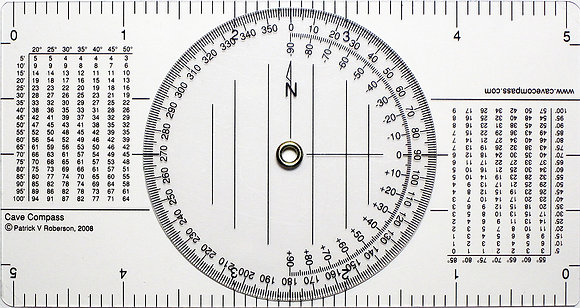# Cave Compass - Standard

\$7.00Price
`Surveying underground can be quite a chore. Why waste time calculating numbers and figuring out angles when the work can be done for you! The Cave Compass gives you the information you need to plot the shot quickly and accurately.`
• Dimensions: 5.25" x 2.75" x . 06"
Wieght: .4 oz (12g)

Standard 10 scale
1"=10', 1"=20', 1"=30', 1"=40', 1"=50'
0-360 compass dial for azimuth
0-90 scale for plotting profile
Corrected distances chart up to 100'